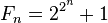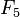# Fermat number facts for kids

Kids Encyclopedia Facts
(Redirected from Fermat prime)

A Fermat number is a special positive number. Fermat numbers are named after Pierre de Fermat. The formula that generates them is$F_{n} = 2^{2^{ \overset{n} {}}} + 1$

where n is a nonnegative integer. The first nine Fermat numbers are (sequence A000215 in OEIS):

F0 = 21 + 1 = 3
F1 = 22 + 1 = 5
F2 = 24 + 1 = 17
F3 = 28 + 1 = 257
F4 = 216 + 1 = 65537
F5 = 232 + 1 = 4294967297 = 641 × 6700417
F6 = 264 + 1 = 18446744073709551617 = 274177 × 67280421310721
F7 = 2128 + 1 = 340282366920938463463374607431768211457 = 59649589127497217 × 5704689200685129054721
F8 = 2256 + 1 = 115792089237316195423570985008687907853269984665640564039457584007913129639937 = 1238926361552897 × 93461639715357977769163558199606896584051237541638188580280321

As of 2007, only the first 12 Fermat numbers have been completely factored. (written as a product of prime numbers) These factorizations can be found at Prime Factors of Fermat Numbers.

If 2n + 1 is prime, and n > 0, it can be shown that n must be a power of two. Every prime of the form 2n + 1 is a Fermat number, and such primes are called Fermat primes. The only known Fermat primes are F0,...,F4.

## Interesting things about Fermat numbers

• No two Fermat numbers have common divisors.
• Fermat numbers can be calculated recursively: To get the Nth number, multiply all Fermat numbers before it, and add two to the result.

## What they are used for

Today, Fermat numbers can be used to generate random numbers, between 0 and some value N, which is a power of 2.

## Fermat's conjecture

Fermat, when he was studying these numbers, conjectured that all Fermat numbers were prime. This was proven to be wrong by Leonhard Euler, who factorised$F_5$ in 1732.Fermat number Facts for Kids. Kiddle Encyclopedia.